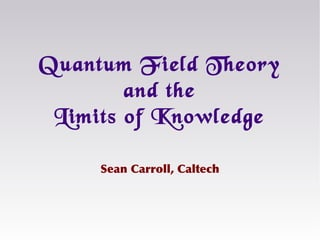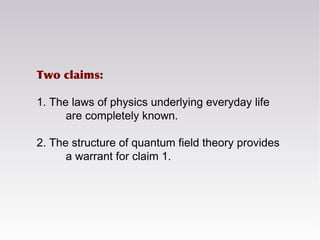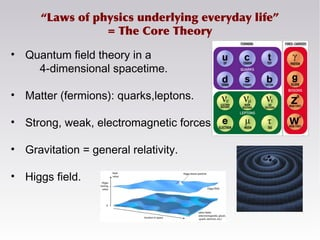Successfully reported this slideshow.

# Quantum Field Theory and the Limits of Knowledge×

# Quantum Field Theory and the Limits of Knowledge

A seminar, given to philosophers, on how quantum field theory allows us to delineate known from unknown in fundamental physics, and why the laws of physics underlying everyday phenomena are known.

A seminar, given to philosophers, on how quantum field theory allows us to delineate known from unknown in fundamental physics, and why the laws of physics underlying everyday phenomena are known.

### Quantum Field Theory and the Limits of Knowledge

1. 1. Sean Carroll, Caltech Quantum Field Theory and the Limits of Knowledge
2. 2. Two claims: 1. The laws of physics underlying everyday life are completely known. 2. The structure of quantum field theory provides a warrant for claim 1.
3. 3. “Laws of physics underlying everyday life” = The Core Theory • Quantum field theory in a 4-dimensional spacetime. • Matter (fermions): quarks,leptons. • Strong, weak, electromagnetic forces. • Gravitation = general relativity. • Higgs field.
4. 4. Long history of embarrassingly premature triumphalism. “[We are] probably nearing the limit of all we can know about astronomy.” – Simon Newcomb, 1888 “The more important fundamental laws and facts of physical science have all been discovered.” – Albert Michelson, 1894 “Physics as we know it will be over in six months.” – Max Born, 1928 There is a 50% chance that “we would find a complete unified theory of everything by the end of the century.” – Stephen Hawking, 1980
5. 5. Perfectly obvious but necessary caveats We’re nowhere close to understanding the fundamental theory of everything. We don’t understand the non-everyday: dark matter, quantum gravity, the Big Bang… We don’t fully understand macroscopic aggregations: condensed matter, chemistry, biology, economics… Quantum mechanics or quantum field theory could always be wrong.
6. 6. Known particles/forces, general relativity (Core theory) Dark matter/energy, new particles/forces, hidden sectors Underlying reality (theory of everything) Higher-level macro-phenomena of everyday life Astrophysics, cosmology
7. 7. The Core Theory in more detail: Quantum Mechanics Think of “configurations,” e.g. the location x of a particle. Assign a complex number to every possible configuration. That describes a quantum state: a “wave function” Ψ(x) that lives in a very-high-dimensional Hilbert space. Schrödinger evolution equation: x x Ψ(x)
8. 8. Measurements in Quantum Mechanics But we don’t “see” the wave function. Measurements return some specific value of the configuration (or other observable). Probability of measurement outcome = |wave function|2 . After measurement, wave function “collapses” (becomes suddenly concentrated on observed outcome). Seems absurd. But – good enough to successfully predict the outcome of every experiment ever done.
9. 9. (Some) Observables are Quantized Standard example: Simple Harmonic Oscillator. Particle moving in a potential , where x is the position and ω is the frequency. Energy is quantized into discrete levels:
10. 10. Quantum Field Theory QFT is not a successor/alternative to QM; it’s just a particular QM model, with a particular Hamiltonian. Namely: “configurations” are “values of (relativistic) fields throughout space.” E.g. φ(x). The quantum state (wave function) is a complex amplitude for each possible field configuration, Ψ[φ(x)]. Examples: electromagnetic field, electron field, top quark field, gravitational field (metric), etc.
11. 11. Particles from fields Each mode acts like a simple harmonic oscillator! Energy levels = number of particles. Wavelength = 1/momentum. Indeed, relativity+QM+particles QFT. Decompose oscillating field into a sum of “modes” of different wavelengths (Fourier transform): = + + …+
12. 12. Interactions Particle interactions are encoded in Feynman diagrams. = + + + …
13. 13. Adding up virtual particles Every particle has a momentum, and total is conserved at each vertex. When there are loops, momentum “flowing through the loop” (q) is arbitrary, and gets summed over. Result is often infinite.
14. 14. don’t need to worry about what happens here Ken Wilson: organize QFT by energy/length scale Remember: energy & momentum ~ 1/(wavelength). IR UV Λ (“cutoff” energy scale) long wavelengths/ low energies short wavelengths/ high energies
15. 15. Think of your theory as only describing energies below the ultraviolet cutoff scale Λ. I.e., only include wavelengths longer than 1/Λ. Result is an effective field theory below Λ. Effective Field Theory
16. 16. All diagrams with N legs contribute to an interaction term (in Lagrangian) between N particles. There are an infinite number of terms in EFT equations of motion… φ4 φ8 φ6
17. 17. Both the field φ and the cutoff Λ have units of energy, and the Lagrangian governing interactions is (energy)4 . So schematically we have: Higher-order terms are negligible at low energy (<< Λ). Only a finite number of relevant/marginal interactions. … but only a finite number of terms matter “relevant” “marginal” “irrelevant”
18. 18. At energies below Λ, an EFT can be a complete theory. Above Λ, new phenomena can kick in. E.g. Fermi theory of weak interactions Standard Model. Effective field theories tell us their regime of applicability: below the ultraviolet cutoff Λ. Fermi coupling
19. 19. “We haven’t quantized gravity,” but I’m treating gravity like a perfectly ordinary effective field theory. Because it is – as long as gravity is weak (far from black holes, Big Bang, etc.). In terms of curvature parameter R, interactions look like Here on Earth, 1st term is 1050 times bigger than 2nd . Quantum Gravity?
20. 20. A given effective field theory with cutoff Λ could have many “ultraviolet completions” at higher energies. That’s why it’s hard to do experiments relevant to quantum gravity: we expect Λ ~ Eplanck ~ 1015 ELHC. Multiple realizability loop quantum gravity string theory dynamical triangulations
21. 21. Known particles/forces, general relativity (Core theory) Dark matter/energy, new particles/forces, hidden sectors Underlying reality (theory of everything) Higher-level emergent phenomena of everyday life Astrophysics, cosmology Underlying physics only influences us via Core Theory.
22. 22. What about new particles/forces? strongly interacting light/ long range/ low energy heavy/ short range/ high energy weakly interacting accessible inaccessible known knowns known unknowns Unknown unknowns = violations of QFT itself.
23. 23. QFT puts very tight constraints on new phenomena. time new particle new interaction If a new particle can interact with ordinary particles: Then that particle can be created in high-energy collisions. “Crossing symmetry.”
24. 24. Constraints on new particles As-yet-undiscovered particles must be either: 1. very weakly interacting, 2. too heavy to create, or 3. too short-lived to detect. In any of those cases, the new particle would be irrelevant to our everyday lives.
25. 25. To be relevant to everyday physics, any new forces must interact with protons, neutrons, electrons, and/or photons. Experiments are ongoing (torsion balances) to search for new, weak, long-range forces. Two ways to hide: 1. weak interactions, or 2. very short ranges. Constraints on new forces
26. 26. Strength(relativetogravity) Range [Long et al. 2003; Antoniadis 2003] Experimental limits on new forces Ruled Out Allowed new gravitational- strength force (10-36 E&M)
27. 27. Known particles/forces, general relativity (Core theory) Dark matter/energy, new particles/forces, hidden sectors Underlying reality (theory of everything) Higher-level emergent phenomena of everyday life Astrophysics, cosmology New particles/forces are too heavy/weak to influence us.
28. 28. gravity other forces matter Higgs quantum mechanics spacetime Punchline: the laws of physics underlying everyday experience. Other phenomena are too massive or weakly-coupled to have any impact on the particles of which we are made.
29. 29. • Astrology is not correct. Implications of the Core Theory • You can’t bend spoons with your mind. • The soul does not survive the body.
30. 30. 3. Accessible deviations from textbook QM. (Hidden variables, spontaneous/induced collapse.) Loopholes? 2. Breakdown of QFT itself. E.g. non-local constraints/ interactions from quantum gravity (holography). 1. New forces with environment-dependent couplings. 4. Divine intervention.
31. 31. Known particles/forces, general relativity (Core theory) Dark matter/energy, new particles/forces, hidden sectors Underlying reality (theory of everything) Higher-level emergent phenomena of everyday life Astrophysics, cosmology